1. Introduction

An operator is a symbol that is used to perform operation with constant and variables. Without an operator, neither an expression .nor a value can be evaluated. So we have seen the functionalities of operators in built in data types only. But it is also possible that an operator can also perform on user-defined data in a manner similar to the built-in data types. C++ provides a mechanism to accomplish this. This mechanism is called as operator overloading.

The mechanism of giving special meaning to an operator is known as operator overloading. Operator overloading is an important feature of Object Oriented Programming in C++. It is so because by using this concept, the programmer would be able to create new definitions to existing operators. It extends the semantics of an operator without changing its syntax. The grammatical rule that governs the use of operator overloading based on the number of operands, precedence and associativity.

• Extending capabilities and functionalities of an operator to operate on user defined data (like object of the class).
• Data or type conversion

2. Operator function

The functionalities of operator overloading is accomplished by a function called operator function. In this function we simply specify what should be done when the operator is applied to the object of the class. The operator function may be a member function or a friend function.

2.1. Defining operator function as member function

When the operator function is defined as a member function, it can be defined either inside or out side the class.

2.1.1. Defining operator function inside the class

The general form of defining the operator function is as:

class class_name

{

private:

//Declare data members

public:

return_type operator_keyword operator_symbol(Argument list)

{

// Operator function body

}

};

2.1.2. Defining operator function outside the class

When the operator function is defined outside the class, its prototype should declared inside the class

The general form of definig operator function outside the class is as:

class class_name

{

private:

//Declare data members

public:

return_type operator_keyword operator_symbol(Argument type);

};

return_type class_name:: operator_keyword operator_symbol(Argument list)

{

// Operator function body

}

2.2. Defining operator function as friend function

When the operator function is defined as a friend function, it is always defined outside the class. However its prototype should be preceded with the keyword friend. It obeys all characteristics of friend function as discussed in chapter 3.

The general form of defining operator function as a friend function is as:

class class_name

{

private:

//Declare data members

public:

friend return_type operator_keyword operator_symbol(argument type);

};

return_type  operator_keyword operator_symbol(argument list)

{

// Operator function body

}

The unary operator function doesn't take any argument when overloaded as a member function and take one argument when overloaded as a friend function.

Program 1

Write a program to overload a unary ++ operator as a member function.

#include<iostream.h>

#include<constream.h>

class demo

{

private:

int rollno;

public:

void getdata();

void operator++();  // Operator function prototype

void display();

};

void demo::getdata()

{

cout<<"Enter rollno: ";

cin>>rollno;

}

void demo::operator++()       // Defining operator function

{

++rollno;

}

void demo::display()

{

cout<<"Rollno = "<<rollno<<endl;

}

main()

{

clrscr();

demo d1;

d1.getdata();

d1.display();

++d1;   //Invoking member function

d1.display();

return(0);

}

Output

Enter rollno: 21

Rollno = 21

Rollno = 22

Explanation

In the above program, the object d1 holds the data value 21 when the function getdata() is invoked. The function display() is invoked to display the value of rollno. Mark that the value of rollno is incremented when the operator function is invoked as ++d1 in the function main(). The operator function is defined outside the class.

Note

The unary operator function may be invoked by the statement as  operator_symbol object_name; or object_name.operator operator_symbol();

In the program1, the operator functioncan be invoked as

++d1;

d1.operator++( ); or

Program 2

Write a program to overload a unary ++ operator as a friend function.

#include<iostream.h>

#include<constream.h>

class demo

{

private:

int rollno;

public:

void getdata();

friend void operator++(demo&); // Prototype for friend operator function

void display();

};

void demo::getdata()

{

cout<<"Enter rollno: ";

cin>>rollno;

}

void operator++(demo &d1)  // Defining operator function as a friend function

{

++d1.rollno;

}

void demo::display()

{

cout<<"Rollno = "<<rollno<<endl;

}

main()

{

clrscr();

demo d1;

d1.getdata();

d1.display();

operator++(d1); //Invoking operator function as a friend function

d1.display();

return(0);

}

Output

Enter rollno: 21

Rollno = 21

Rollno = 22

Explanation

In the above program, the object d1 holds the data value 21 when the function getdata() is invoked. The function display() is invoked to display the value of rollno. Mark that the value of rollno is incremented when the operator function as friend is invoked as operator++(d1) in the function main(). The operator function is defined outside the class because it is declared as a non-member or friend function. We have used pass by reference method to see the change of content in the object d1..

Note

When the unary operator function is invoked as a friend function, then it may take the form as   operator_symbol object_name; or operator operator_symbol(object_name); for invoking the function.

In the program2, the operator function can be invoked as

++d1;

operator++(d1 );

or

The binary operator function takes one argument when overloaded as a member function and take two arguments when overloaded as a friend function.

Program 3

Write a program to overload a binary + operator as a member function

#include<iostream.h>

#include<constream.h>

class demo

{

private:

int a;

int b;

public:

void getdata();

demo operator+(demo);

void display();

};

void demo::getdata()

{

cout<<"Enter two values: ";

cin>>a>>b;

}

demo demo::operator+(demo d2)

{

demo res;

res.a=a+d2.a;

res.b=b+d2.b;

return(res);

}

void demo::display()

{

cout<<"a = "<<a<<endl;

cout<<"b = "<<b<<endl;

}

main()

{

clrscr();

demo d1,d2,d3;

cout<<"Object d1"<<endl;

d1.getdata();

cout<<"Object d2"<<endl;

d2.getdata();

d3=d1.operator+(d2);

cout<<"Contents of object d1.."<<endl;

d1.display();

cout<<"Contents of object d2.."<<endl;

d2.display();

cout<<"Contents of object d3.."<<endl;

d3.display();

return(0);

}

Output

Object d1

Enter two values: 10

20

Object d2

Enter two values: 30

40

Contents of object d1..

a = 10

b = 20

Contents of object d2..

a = 30

b = 40

Contents of object d3..

a = 40

b = 60

Explanation

Let us have a look to the operator function

demo demo::operator+(demo d2)

{

demo res;

res.a=a+d2.a;

res.b=b+d2.b;

return(res);

}

Here the function will add two values of  a and b in both object d1 and d2 . The resultant values will be assigned to res, and it will return to d3 as the function is invoked as d3=d1.operator+(d2); in function main().Here the the data member of object d1 are accessed directly, whereas the data members of object d2 are accessed using the dot operator through argument in the operator function. It can be represented in the following figure 1 as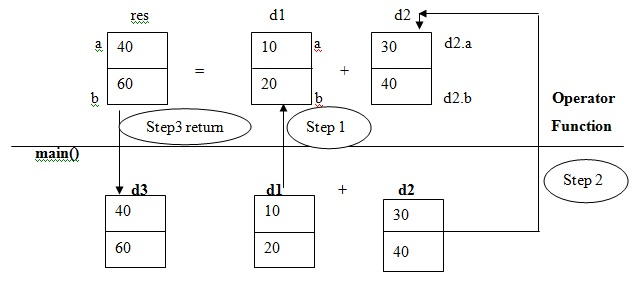It is a rule for overloading binary operator that the content of first operand (d1) is accessed directly, whereas the content of second operand (d2) is accessed explicitly using dot operator.

Note

When the binary operator function is invoked as a member function, then it may take the form as   object1 perator_symbol object2; or object1.operator operator_symbol(object2); for invoking the function.

In the program3, the operator function can be invoked as

d3= d1 + d2;

d3= d1.operator+(d2 );

or

Program 4

Write a program to overload a binary + operator as a friend function

#include<iostream.h>

#include<constream.h>

class demo

{

private:

int a;

int b;

public:

void getdata();

friend demo operator+(demo,demo);

void display();

};

void demo::getdata()

{

cout<<"Enter two values: ";

cin>>a>>b;

}

demo operator+(demo d1,demo d2)

{

demo res;

res.a=d1.a+d2.a;

res.b=d1.b+d2.b;

return(res);

}

void demo::display()

{

cout<<"a = "<<a<<endl;

cout<<"b = "<<b<<endl;

}

main()

{

clrscr();

demo d1,d2,d3;

cout<<"Object d1"<<endl;

d1.getdata();

cout<<"Object d2"<<endl;

d2.getdata();

d3=operator+(d1,d2);

cout<<"Contents of object d1.."<<endl;

d1.display();

cout<<"Contents of object d2.."<<endl;

d2.display();

cout<<"Contents of object d3.."<<endl;

d3.display();

return(0);

}

Output

Object d1

Enter two values: 10

20

Object d2

Enter two values: 30

40

Contents of object d1..

a = 10

b = 20

Contents of object d2..

a = 30

b = 40

Contents of object d3..

a = 40

b = 60

Explanation

Here the function will add two values of  a and b in both object d1 and d2 . The resultant values will be assigned to res, and it will return to d3 as the function is invoked as d3=operator+(d1,d2); in function main().Here the data members of object d1 and the data members of object d2 are accessed using the dot operator through argument in the operator function.

Note

When the binary operator function is invoked as a friend function, then it may take the form as   object1 perator_symbol object2; or operator operator_symbol(object1, object2); for invoking the function.

In the program4, the operator function can be invoked as

d3=d1 + d2;

d3= operator+(d1, d2 );

or

Overloading of unary increment (++) and decrement operators impose no difference as prefix or postfix operation. The compiler doesn't differentiate this purpose whether these operators could be overloaded as prefix or postfix. A proposed syntax is used to differentiate this as given in the following prototypes like

operator++(int); used for postfix operation

operator++(); used for prefix operation

No other type can be specified as argument for postfix purpose except the int type. However this operator can be used for all types like float, double etc.

Program 5

Write a program to overload unary ++ and - as prefix and postfix operator

#include<iostream.h>

#include<constream.h>

class example

{

private:

int a;

float b;

public:

example()

{

a=2;

b=2.5;

}

void operator++(int);

void operator++();

void display();

};

void example::operator++(int)

{

a++;

b++;

}

void example::operator++()

{

++a;

++b;

}

void example::display()

{

cout<<"a = "<<a<<endl;

cout<<"b = "<<b<<endl;

}

main()

{

clrscr();

example e1;

cout<<"Initial values.."<<endl;

e1.display();

e1++; //invoking for postfix increment

cout<<"After postfix increment.."<<endl;

e1.display();

++e1; //invoking for prefix increment

cout<<"After postfix increment.."<<endl;

e1.display();

return(0);

}

Output

Initial values..

a = 2

b = 2.5

After postfix increment..

a = 3

b = 3.5

After postfix increment..

a = 4

b = 4.5

Explanation

In the above program, the operator ++ is overloaded as both prefix and postfix use. It is to be noted that if the operator is overloaded as both postfix and prefix use without an (int) as argument, it will display a warning message. However to avoid this we have used (int) as parameter for postfix evaluation.

There are certain rules are followed during the implementation of operator overloading. Some of them are summarized here.

§  Only existing operators are overloaded that are supported by C++.

§  We cannot the basic meaning of an operator. That means a plus (+) sign cannot be used for multiplication.

§  Following operators cannot be overloaded

 Operators Meaning sizeof() Size of operator . Membership operator .* Pointer to member operator :: Scope resolution operator ?: Conditional operator

§  Following operators cannot be overloaded as friend function

 Operators Meaning = Assignment operator () Function call operator [] Subscripting operator -> Member access operator

§  The Unary operator function takes no explicit argument and returns nothing when overloaded as a member function. But when overloaded by means of a friend function take one argument as reference object of the concerned class.

§  The binary operator function takes one explicit argument when overloaded as a member function and take two explicit arguments when overloaded as a friend function.

6. Type Conversion

Type conversion refers to the process of conversion of one data type to another type. This can be achieved so easily in conventional C language for basic data types. However we have to write certain conversion routine to perform type conversion for user defined types.

In C++ the type conversion arises in three categories as

1)      Conversion from basic to class type

2)      Conversion from class type to basic type

3)      Conversion from one class type to another class type

As the compiler does not support automatic type conversion for the user defined data types, so we extensively use constructor and casting operator function in source and destination classes respectively. The following table summarizes this.

Table 1. Type Conversion

 Conversion Type Source Class Destination Class Basic -> Class × Constructor Class -> Basic Casting operator function × Class -> Class Casting operator function Constructor

The conversion from a class to any other type should make use of casting operator function in the source class.

The conversion from any other type to class type requires a constructor to be used in destination class.

Note:

Remember that whenever a conversion using constructor is performed in the destination class, we should be able to access the data members of the object sent by the source class as an argument. We must use special access function to see that the private data of source class could flow to the destination class.

6.1. Conversion from basic to class type

Here we use a constructor in the class so that the object can access the basic data type value and assign to its specific data member. During invoking this kind of constructor, the left hand side of assignment operator is a class type(an object) and right

Program 6

Write a program to convert a basic type to class type

#include<iostream.h>

#include<constream.h>

class conversion

{

private:

int a;

float b;

public:

conversion()

{

a=10;

b=2.5;

}

conversion(float x)//constructor for conversion

{

b=x;

}

void display()

{

cout<<"a = "<<a<<endl;

cout<<"b = "<<b<<endl;

}

};

main()

{

clrscr();

conversion c1;

c1.display();

c1=3.15; // Converting basic type to class type

c1.display();

return(0);

}

Output

a = 10

b = 2.5

a = 21

b = 3.15

Explanation

In the above program the object c1 , the default constructor is invoked and initializes a =10 and b=2.5 respectively as shown below.

Object c1

a =10

b=2.5

Look at the statement c1=3.15, in which the left hand side is a class type and right hand side is a basic type. This statement will invoke the constructor with one argument in the class conversion (which is a destination class) as shown below.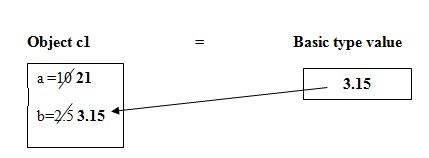The value of a is again initialized to 21 and 3.15 is a float type which is assigned to data member b in the class type (c1).

6.2. Conversion from class to basic type

To perform this kind of conversion C++ allows us to define a casting operator function to convert a class type data to a basic type. In this function , the compiler searches for the keyword operator followed by the data type. The general form of a casting operator function is as:

operator typename()

{

//Statements

}

In the above syntax, the typename is always a basic type. For example, the operator float() convets a class type to float type.

The casting operator should satisfy the following constraints.

1)      It must be a member function

2)      It must not have any argument

3)      It must not have return type

During this conversion, the left hand side is a basic type and the right hand side is a class type.

Program 7

Write a program to convert a class type data to basic type

#include<iostream.h>

#include<constream.h>

class conversion

{

private:

int a;

float b;

public:

conversion()

{

a=10;

b=2.5;

}

operator int()

{

return(a);

}

operator float()

{

return(b);

}

void display()

{

cout<<"a = "<<a<<endl;

cout<<"b = "<<b<<endl;

}

};

main()

{

clrscr();

conversion c1;

int x;

float y;

x=c1; // operator int() is invoked

y=c1; // operator float() is invoked

cout<<"x = "<<x<<endl;

cout<<"y = "<<y<<endl;

return(0);

}

Output

x = 10

y = 2.5

Explanation

In the above program the data members are initialized as a=10 and b=10 when the object c1 is declared and the default constructor is invoked. The variables x and y are of basic types declared in function in main(). Our motto is to assign the value of a to x and that of b to y. So for this let us look at the statement x=c1 and y=c1 in the following figure as: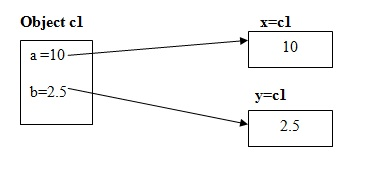It is clear from the above figure that  when the statement x=c1 is executed, the operator int() function is invoked and when the statement y=c1 is executed the function operator float() is invoked. So the int value is returned to x and float value to y respectively.

6.3. Conversion from class to class type

This type of conversion can be done in two ways. One is to declare a conversion function in the source class or a constructor with one argument in the destination class. Let us see the both methods in the following program 8.

Program 8

Write a program to convert one class type to another type

Method 1

#include<iostream.h>

#include<constream.h>

class A

{

private:

int code;

int item;

float amt;

public:

A()

{

code=111;

item=5;

amt=100.0;

}

void display()

{

cout<<"Item Code   = "<<code<<endl;

cout<<"No of items ="<<item<<endl;

cout<<"Amount      = "<<amt<<endl;

}

int getcode()

{

return(code);

}

int getitem()

{

return(item);

}

float getamt()

{

return(amt);

}

};

class B

{

private:

int code;

float amt;

public:

void display()

{

cout<<"Item Code   = "<<code<<endl;

cout<<"Amount      = "<<amt<<endl;

}

B(A a1)

{

code=a1.getcode();

amt=a1.getamt();

}

};

main()

{

clrscr();

A a1;

B b1=a1;

cout<<"Product details of class A "<<endl;

a1.display();

cout<<"Product details of class B "<<endl;

b1.display();

return(0);

}

Output

Product details of class A

Item Code       = 111

No of items     =5

Amount          = 100

Product details of class B

Item Code       = 111

Amount          = 100

Explanation

In the above program in method 1, class A contains three data members like code, item and amt which is a source class. The class B contains two data members like code and amt, which will be assigned from class A object(a1) to class B object (b1) by the statement B b1=a1 in the function main( ).This can be illustrated in the following figure as: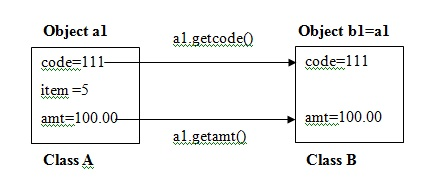Here the destination class B contains the constructor that takes object a1 of class A as an argument to access the data member code and amt from the class A to class B.

Method 2 (Alternative of Program 8)

#include<iostream.h>

#include<constream.h>

class A

{

private:

int code;

int item;

float amt;

public:

A()

{

code=111;

item=5;

amt=100.0;

}

void display()

{

cout<<"Item Code   = "<<code<<endl;

cout<<"No of items ="<<item<<endl;

cout<<"Amount      = "<<amt<<endl;

}

operator float()

{

return(amt);

}

operator int()

{

return(code);

}

};

class B

{

private:

int code;

float amt;

public:

void display()

{

cout<<"Item Code   = "<<code<<endl;

cout<<"Amount      = "<<amt<<endl;

}

B(int x, float y)

{

code=x;

amt=y;

}

};

main()

{

clrscr();

A a1;

int x;

float y;

x=a1;

y=a1;

B b1(x,y);

cout<<"Product details of class A "<<endl;

a1.display();

cout<<"Product details of class B "<<endl;

b1.display();

return(0);

}

Output

Product details of class A

Item Code       = 111

No of items     =5

Amount          = 100

Product details of class B

Item Code       = 111

Amount          = 100

Explanation

In the above program in method 2, class A contains three data members like code, item and amt which is a source class. The class B contains two data members like code and amt, which will be assigned from class A object (a1) to class B object (b1) .This can be illustrated in the following figure as: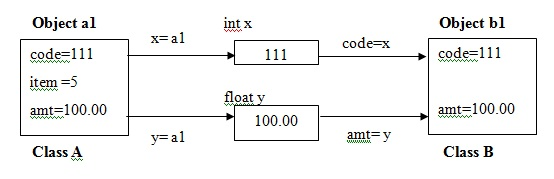Here the source class A contains two casting operator functions that return the data member code and amt to two variables x and y respectively. The values of x and y are passed as argument to the constructor in the destination class B to assign the value of x and y to its data members code and amt respectively.

We know that the insertion (<<) operator and extraction operator (>>) are used with cin and cout object to perform input and output operation. It is also possible to overload these operators with the help of friend function. Remember that cin is an object of istream class and cout is an object of ostream class.

friend ostream & operator <<(ostream &out, obj)

{

// Statements

}

In the above declaration, out is an output stream object like cout and obj is a user defined  class object.

Program 9

Write a program to overload the insertion operator

#include<iostream.h>

#include<constream.h>

class demo

{

private:

int rollno;

public:

demo()

{

rollno=21;

}

friend ostream & operator <<(ostream &out, demo d1)

{

out<<"Roll no : "<<d1.rollno;

return out;

}

};

main()

{

clrscr();

demo d;

cout<<d;

return(0);

}

Output

Roll no : 21

Explanation

Here the overloaded insertion operator allows us to display the content of object d through cout statement as cout<<d.

friend istream & operator <<(istream &in, obj)

{

// Statements

}

In the above declaration, in is an input stream object like cin and obj is a user defined  class object.

Program 10

Write a program to overload the extraction operator

#include<iostream.h>

#include<constream.h>

class demo

{

private:

int rollno;

public:

demo()

{

rollno=21;

}

friend istream & operator >>(istream &in, demo d1)

{

cout<<"Enter rollno :";

in>>d1.rollno;

return in;

}

};

main()

{

clrscr();

demo d;

cin>>d;

return(0);

}

Output

Enter rollno :21

Explanation

Here the overloaded extraction operator directly used with cin statement to store the value for data member in the class object.

8. The this pointer

The keyword this is a pointer which stores the memory address of the object of the class in question. The this pointer is implicitly defined in each member function (public or private). Every member function is born with a pointer called this which points to the object with which the member function is associated. Using this pointer any member function can find out the address of the object of which it is a member. The this pointer is always named as this and is a hidden argument passed to every member function as if the member function declared as shown below:

void demo::display( demo* this);

Program 11

Write a program to display the address of the object using this pointer

#include<iostream.h>

#include<constream.h>

class demo

{

public:

void display()

{

}

};

main()

{

clrscr();

demo d;

d.display();

return(0);

}

Output

Explanation

From the above output it is clear that every member function contains this pointer to hold the address of the object in question. Remember that this pointer is not accessible by any non-member function.

Program 12

Write a program to display the data values using this pointer

#include<iostream.h>

#include<constream.h>

class employee

{

private:

int emp_id;

char name;

float salary;

public:

void getdata()

{

cout<<"Enter emp id, name and salary :";

cin>>emp_id>>name>>salary;

}

void display()

{

cout<<"Employee id :"<<this->emp_id<<endl;

cout<<"Name      :"<<this->name<<endl;

cout<<"Salary    :"<<this->salary<<endl;

}

};

main()

{

clrscr();

employee e1;

e1.getdata();

e1.display();

return(0);

}

Output

Enter emp id, name and salary :12104

Samir

7800

Employee id :12104

Name       :Samir

Salary     :7800

Explanation

As the pointer this holds the memory address of the object e1, so it access data member using the member access operator(->) like this->emp_id, this->name and this-> salary in display() function.

Programming Examples

Program 12

Write a program to overload an assignment operator = to assign the content of one object to another object of the same class.

#include<iostream.h>

#include<constream.h>

class A

{

private:

int x;

float y;

public:

A(int, float);

void operator =( A obj);

void display();

};

A :: A(int one,float two)

{

x=one;

y=two;

}

void A ::operator =(A a2)

{

x=a2.x;

y=a2.y;

}

void A :: display()

{

cout<<"x = "<<x<<endl;

cout<<"y = "<<y<<endl;

}

main()

{

clrscr();

A a1(20,-30.25);

A a2(30,40.25);

cout<<"Content of 1st object"<<endl;

a1.display();

cout<<"Content of 2nd object"<<endl;

a2.display();

a1=a2;

cout<<"Now content of 1st object"<<endl;

a1.display();

return(0);

}

Output

Content of 1st object

x = 20

y = -30.25

Content of 2nd object

x = 30

y = 40.25

Now content of 1st object

x = 30

y = 40.25

Explanation

Here the operator function makes all necessary assignments from the content of second object(a2) to the first object (a1). This is done on executing the statement as a1=a2 in the main() function. The same statement can also be stated as a1.operator(a2).

Program 13

Write a program to overload relational operator < and == to compare two dates.

#include<iostream.h>

#include<constream.h>

class date

{

private:

int dd;

int mm;

int yy;

public:

void getdata();

int date::operator==(date &d);

int operator<(date &);

void display();

};

void date::getdata()

{

cin>>dd>>'-'>>mm>>'-'>>yy;

}

int date::operator==(date &d)

{

return(this->mm==d.mm && this->dd==d.dd && this->yy==d.yy);

}

int date::operator<(date &d)

{

if(this->yy==d.yy)

{

if(this->mm==d.mm)

return(this->dd < d.dd);

else

return(this->mm < d.mm);

}

return(this->yy < d.yy);

}

void date::display()

{

cout<<dd<<'-'<<mm<<'-'<<yy;

}

main()

{

clrscr();

date d1,d2;

cout<<"Enter 1st date as dd-mm-yy format :";

d1.getdata();

cout<<"Enter 2nd date as dd-mm-yy format :";

d2.getdata();

if(d1<d2)

{

d1.display();

cout<<" is less than ";

d2.display();

}

if(d1==d2)

{

d1.display();

cout<<" is equal to ";

d2.display();

}

return(0);

}

Output 1

Enter 1st date as dd-mm-yy format :1-11-2011

Enter 2nd date as dd-mm-yy format :11-11-2011

1-11-2011 is less than 11-11-2011

Output 2

Enter 1st date as dd-mm-yy format :11-11-2011

Enter 2nd date as dd-mm-yy format :11-11-2011

11-11-2011 is equal to 11-11-2011

Explanation

In the above program, the date class has two overloaded relational operators : the less than (<) and equal to(==). The main() function declares two dates, compares them and displays the following messages:

1-11-2011 is less than 11-11-2011

11-11-2011 is equal to 11-11-2011

Program 14

Write a program to overload [ ] operator to display the element of an array

#include<iostream.h>

#include<constream.h>

class array

{

private:

int a;

public:

array()

{

a=10;

a=20;

a=30;

a=40;

a=50;

}

int operator[](int i)

{

return(a[i]);

}

};

main()

{

clrscr();

array a1;

cout<<a1;

return(0);

}

Output

30

Explanation

In the above program, the class array declares an array of five integers. The constructor function initializes the array elements as 10,20,30,40 and 50 respectively. The operator function returns the value of the array as indexed by the value of its parameter. So it displays the value at index 2 as 30 on the output screen.

Program 15

Write a program to overload [ ] operator to modify the element of an array

#include<iostream.h>

#include<constream.h>

class array

{

private:

int a;

public:

array()

{

a=10;

a=20;

a=30;

a=40;

a=50;

}

int &operator[](int i)

{

return(a[i]);

}

};

main()

{

clrscr();

array a1;

a1=45;

cout<<a1;

return(0);

}

Output

45

Explanation

To change the value of the array, we can specify the return value of operator[] () function as a reference. In this program, the operator[]() function returns a reference to the array element indexed by i such that it can be used on the left hand side of the assignment operator to modify the element of the array from 30 to 4

Program 16

Write a program to overload a ( ) operator

#include<iostream.h>

#include<constream.h>

class mark

{

private:

int m1;

int m2;

public:

mark()

{

m1=65;

m2=75;

}

void display()

{

cout<<"Mark1 = "<<m1<<endl;

cout<<"Mark2 = "<<m2<<endl;

}

mark operator()(int p, int q)

{

m1=p;

m2=q;

return(*this);

}

};

main()

{

clrscr();

mark mobj;

cout<<"Initial marks...."<<endl;

mobj.display();

cout<<"New marks...."<<endl;

mobj.operator()(89,99);

mobj.display();

return(0);

}

Output

Initial marks....

Mark1 = 65

Mark2 = 75

New marks....

Mark1 = 89

Mark2 = 99

Explanation

The above program uses the overloaded operator() function to assign the value of its two arguments to m1 and m2 of the object mobj to which it is pointed. When we use the ( ) operator in the program, the object  (mobj)  that generates the call is pointed to by the this pointer.

Remember that when we overload the ( ) operator function, it means that we are creating a new way to call a function with an arbitrary number of parameters. We can use any type of parameters and return type of value. We can also specify default arguments.

Program 17

#include<iostream.h>

#include<constream.h>

class example

{

public:

int var1;

example *operator->()

{

return(this);

}

void display()

{

cout<<"Var1 = "<<var1<<endl;

}

};

main()

{

clrscr();

example e1;

e1->var1=21;

e1.display();

return(0);

}

Output

Var1 = 21

Explanation

In the above program we initialize the value of var1 through the statement e1->var1=21. The operator ->() function returns the this pointer to the object e1. Here the object e1 makes the call to the operator->( ) function.

Remember that the operator->( ) function must return a pointer to an object of the class that the function operates upon. The data element (here var1) must be some member accessible within the object (here e1).

Summary

• Operator overloading is one of the meaningful concept in C++ language that plays an important to solve real life problems.
• We can use the same syntax just as basic data types for user defined data types such as objects of the relevant class.
• Almost all operators of C++ can be overloaded except those mentioned in section
• The operator overloading can be accomplished by a special function known as operator function.
• The operator function must either be non-static member function or friend functions.
• The compiler does not support automatic type conversion; however we need to write the conversion function to achieve type conversion for user defined data types.
• The conversion function should posses the following rules
1. It should be a member function
2. It should not have any argument.
3. It should not specify any return type.

Review Questions

2)      What is an operator function?

3)      How can we overload unary and binary operators? Explain each of them briefly.

4)      How do we overload stream operators? Explain with suitable examples.

6)      What is a conversion function? Discuss its rule.

7)      How can you overload [] and ( ) operator. Explain with suitable example.

8)      How can you overload -> operator?

9)      What are the mechanism of overloading prefix and postfix unary operators.